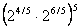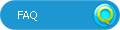# HotmathTitle:
Hotmath
Author:
Hotmath

Problem: 1

Rewrite using rational exponent notation.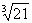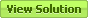Problem: 3

Rewrite using rational exponent notation.Problem: 5

Rewrite using rational exponent notation.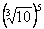Problem: 7

Rewrite using rational exponent notation.Problem: 15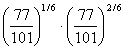Problem: 17

Simplify.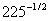Problem: 19

Simplify.Problem: 21

Simplify.Problem: 23

Simplify.Problem: 25

Simplify.Problem: 27

Simplify.Problem: 29

Simplify.Problem: 31

Simplify.Problem: 33

Write using positive exponents, with a rational denominator.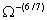Problem: 35

Simplify.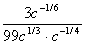Problem: 37

Evaluate without using a calculator.Problem: 39

Evaluate without using a calculator.Problem: 41

Evaluate without using a calculator.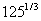Problem: 43

Evaluate without using a calculator.Problem: 45

Evaluate without using a calculator.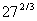Problem: 47

Evaluate without using a calculator.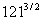Problem: 49

Evaluate without using a calculator.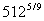Problem: 51

Evaluate without using a calculator.Problem: 55

Evaluate.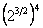Problem: 59

Evaluate.